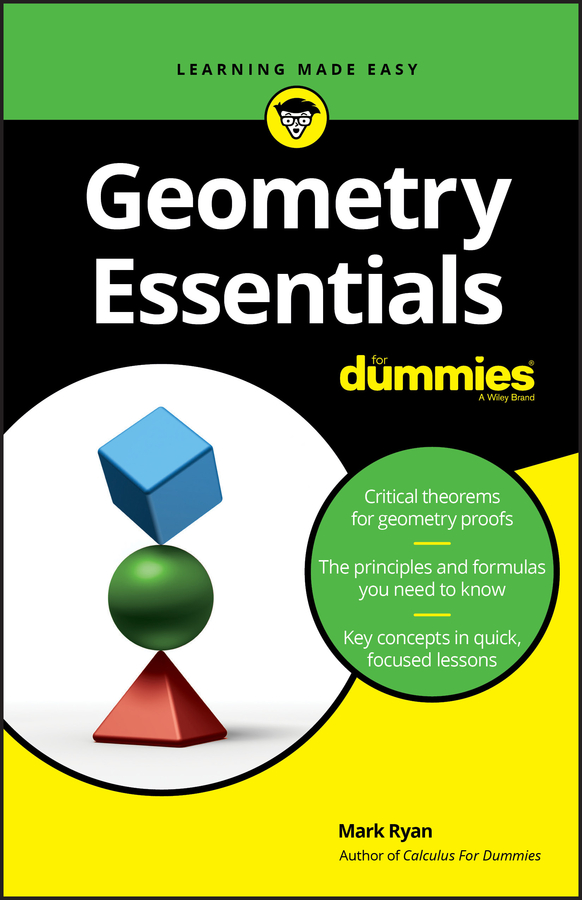##### Geometry Essentials For DummiesA hyperbola is the set of all points in the plane such that the difference of the distances from two fixed points (the foci) is a positive constant. Hyperbolas always come in two parts, and each one is a perfect mirror reflection of the other. There are horizontal and vertical hyperbolas, but regardless of how the hyperbola opens, you always find the following parts:

• The center is at the point (h, v).

• The graph on both sides gets closer and closer to two diagonal lines known as asymptotes. The equation of the hyperbola, regardless of whether it's horizontal or vertical, gives you two values: a and b. These help you draw a box, and when you draw the diagonals of this box, you find the asymptotes.

• There are two axes of symmetry:

• The one passing through the vertices is called the transverse axis. The distance from the center along the transverse axis to the vertex is represented by a.

• The one perpendicular to the transverse axis through the center is called the conjugate axis. The distance along the conjugate axis from the center to the edge of the box that determines the asymptotes is represented by b.

• a and b have no relationship; a can be less than, greater than, or equal to b.

• You can find the foci by using the equation f 2 = a2 + b2.